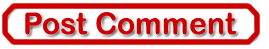Best of the Week# The Bizarre Mathematics Of How Negative Interest Rates Create Stratospheric Profits

Stock-Markets / Quantitative Easing Jul 17, 2020 - 01:34 PM GMT

By: Dan_Amerman

There is an increasingly good chance that the United States could end up following Europe and Japan, and that the Federal Reserve could use its vast powers of monetary creation to force a move to negative interest rates.

If that deeply unnatural event happens, it will invert and distort the very foundations of investment pricing, in ways that are little understood by most investors today.

It will also - for a time - create an unnatural source of profits that most investors have no idea about, because it has never happened before in the United States (and is still in the early stages in the United Kingdom).

Why should anyone care? Here are two reasons, as explored in this analysis:

1) The Federal Reserve using monetary creation to purchase bonds at negative interest rates would - for a time - mathematically unlock a deeply unnatural source of profits. This source simply does not exist with positive interest rates - but is more powerful than compound interest.In the graphic above, the height of the purple bars represents the profits associated with one of the largest and longest running bull markets in bonds in U.S. history. As can be readily seen with the golden bars, those profits could be dwarfed by a move to negative interest rates.

2) Another bizarre side effect of the mathematics of negative interest rates is that those profits are unlocked instantly. So the inversion of the natural financial order that is negative interest rates could lead to the unnatural result of an investor making more money in a matter of weeks than they could ordinarily earn in decades with compound interest, all else being equal.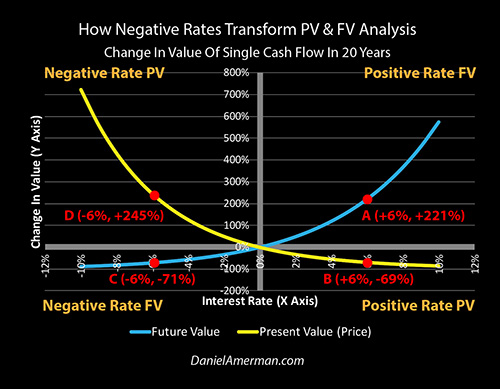To understand how this could be, we will develop the XY graphic above. The right side of the graph, positive interest rates, is all of our experience with what most of us likely thought was the only natural and possible investment world, where wealth is steadily created over time by rational investors acting in their own self-interests.

When we cross the zero percent barrier and go to the left side, we find the twisted, inverted, unnatural world of negative interest rates, where the rational financial rewards for long term investing are replaced by irrational long term and unrelenting pain for many savers and investors - even while a new form of stunning potential profits can leap forward in time.

This analysis is part of a series of related analyses, which support a book that is in the process of being written. Some key chapters from the book and an overview of the series are linked here.

### Creating Future Wealth

For the understanding of how these extraordinary profits are created we need to go to the bedrock fundamentals of financial analysis, as professionals in the field usually learn when they are in college, but which many individual investors are not fully aware of. However, while they are based on that common foundation - negative rates lead to an inversion of reality that is so complete that even professionals with decades of experience can have trouble understanding the bizarre ways negative rates change investment returns and prices.

This means that we can't just look to the past and project it forward, which is what most people do when they try to make future investment plans. The issue is that this has never happened in the U.S. markets, and the profits are on the front end. Once it has already happened, for those who wait until then to learn - it may be too late.

Instead we need to go to the underlying fundamentals, which is why I wrote chapters 10, 11 and 12 in the free book. If you do have those chapters, it may be worth going back and reviewing them, otherwise I will do my best to explain the basics in a very compressed manner herein, so we can get to the implications for negative rates.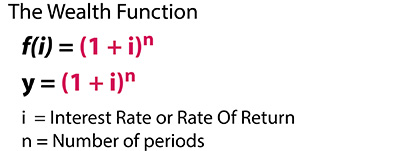Underlying everything else when it comes the field of finance is a basic function that I call the wealth function. This is how future returns for investments are calculated, and it is also the foundation for how investment prices are calculated today. This is particularly true for bonds, but it is also the foundation underlying rational stock and real estate pricing. (As well as why bond, stock, and real estate pricing levels have all fundamentally changed as the Fed has forced every lower interest rates on the nation.)Now if we take this wealth function as explored in detail in Chapter 10, and we multiply it times how much money we have to invest today then we get the "future value", how much money we will have in the future if we invest money today at a given rate for given period of time. The most common term for this with the general public is compound interest, but the formula applies to a lot more than just interest payments.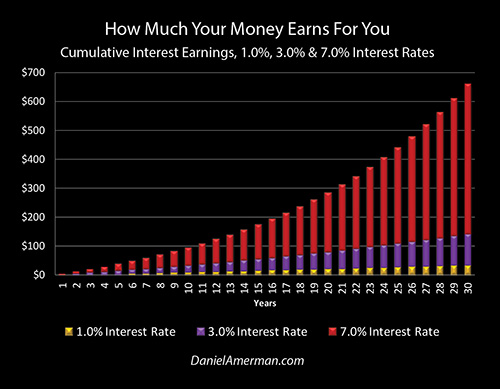How it works is illustrated above. What we have is an exponential function that grows much stronger with higher rates, and longer periods of time. For instance, if we look at the average yield for 10 year Treasuries from 1962 to 2000, that was just over 7%, and if we put that into our future value formula for 30 years, that shows that we will earn \$661 in interest over 30 years, as shown with the rightmost red bar.

(There is no need to actually run any numbers or use any equations to follow this analysis, I will do all of that and illustrate it for you. That said, for those who wish, I'm keeping the examples simple enough - even the esoterics of negative rate price changes - so that the numbers can easily be run and explored on your own using any spreadsheet, hand calculator or even with the calculator app on your phone. As an example, using the future value (FV) formula of ((1 + i) ^ n), ((1 + .07) ^ 30 = 7.61, with a starting investment of \$100 that would mean a future value of \$761 at a 7% rate over 30 years, of which \$100 would be the return of the investment, and \$661 would be interest earnings.)

The purple bars are compound interest at a 3% interest rate, and over 30 years those would be \$143. This much lower interest rate is more in the range of what we have experienced since the Federal Reserve began implementing its aggressive low interest rate polices in 2001, and shows that the cost for investors is a 78% reduction in the ability to build wealth over the long term using the reliable "magic" of compound interest.

(The numbers are ((1 + i) ^ n), (1 + .03) ^ 30) = 2.43, \$100 X 2.43 = \$243, \$243 - \$100 = \$143 in future interest earnings.)

The golden bars are compound interest at a 1% interest rate, and over 30 years those would be \$35. This is more comparable to what long term government bond rates have been since the Fed slammed short term interest rates down to 0% in the attempt to contain the economic and market damage from the pandemic and shutdowns. So the price of the Federal Reserve's extreme measures - which they are currently pledging to maintain until at least 2022 - is a 95% destruction of the ability for investors to create wealth over the long term via compound interest.

(The numbers are ((1 + i) ^ n), (1 + .01) ^ 30) = 1.35, \$100 X 1.35 = \$135, \$135 - \$100 = \$35 in future interest earnings.)

This ties in very closely to what we went through in Chapter 17 (link here) which is the true cost of the national debt for investors and that is the catastrophic collapse of the ability to reliably create wealth through compound interest over time. This is a pervasive problem for all savers that is likely to only grow worse with the current explosion in the national debt.

### Profits (Or Losses) Today

The next step, as explored in Chapter 11, is to use the wealth function to travel the other direction in time, and find the price we will pay today for a given cash flow in the future (i.e. the present value). This is tremendously useful because it tells us exactly how much money we can make - or lose - if interest rates change, and understanding how this works is the key to understanding the counterintuitive ways in which negative interest rates can create extraordinary profits with blinding speed, and with a power that can even exceed that of compound interest.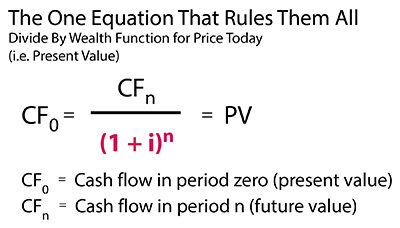As shown in the formula, to get price today we take the inverse of the future value. We divide by the wealth function instead of multiplying by it.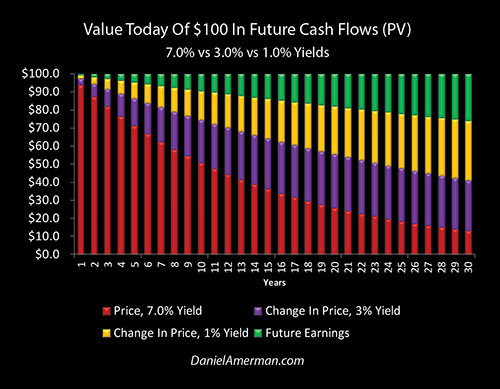This inverse relationship is fairly simple, once we step back and think about it. We've already established that \$100 would become \$761 in 30 years at a 7% rate and with compound interest, so we know the ratio is that 1 becomes 7.61. To lock in that 7% rate today, on a single \$100 cash flow that we will receive 30 years in the in the future (such as a zero coupon bond), we would have to divide by that ratio instead of multiplying by it, meaning we would have to pay 1 / 7.61, which is 13.14%. So we pay a price of \$13.14 today, for \$100 we will receive in 30 years, as shown with red bar on the right. We take that \$13.14, multiply times 7.61, and sure enough, we get \$100 and have locked in the 7% yield - and the compound interest on it - over 30 years, so long as we hold that investment until it matures.

(The math is one notch more complicated here because we are calculating the inverse, but is still easily doable on your phone or calculator. The numbers are 1 / ((1 + i) ^ n), 1 / ((1 + .07) ^ 30)), 1 / 7.61 = 13.14%, \$100 X 13.14% = a dollar price of \$13.14 today.)

The purple bars show the prices we would pay in each year to lock in a 3% compounded rate of return. Again, we have already established the ratio, and we know that \$100 becomes \$243 over 30 years at a 3% rate with compound interest. So we divide 1 by 2.43, which is 41.15%, and the price we would pay today for that \$100 in the future is \$41.15. Again, we can test this by multiplying the \$41.15 times 2.43, and getting the \$100 in the future.

(The numbers are 1 / ((1 + i) ^ n), 1 / ((1 + .03) ^ 30)), 1 / 2.43 = 41.15%, \$100 X 41.15% = a dollar price of \$41.15 today.)

The next step is that if we had bought the future cash flow at a 7% yield, and we were to sell it at a 3% yield (same day for this simplified example), then we would earn a huge profit of 213% on our investment (\$41.15 / \$13.14 = 313%, of which 213% is profit).

Crucially, as we get closer to zero percent interest rates - the price gains get larger, We had already established \$100 becomes only worth \$135 if invested at 1% over 30 years. This is true not only because of the lower rate itself, but because compound interest (aka exponential compounding) becomes much weaker at lower interest rates. To get the price today then, we divide 1 by 1.35, dividing by that much smaller number produces a much larger price, that of 74.07%, and that means we would pay \$74.07 for a cash flow 30 years out, as can be seen by the height of the golden bar on the right.

(The numbers are 1 / ((1 + i) ^ n), 1/((1+.01)^30)), 1 / 1.35 = 74.07%, \$100 X 74.07% = a dollar price of \$74.07 today.)

So moving interest rates the 4 percentage points from 7% to 3% produced a price gain of \$28.01 (\$41.15 - \$13.14). But it only took half of that, a 2 percentage point change from 3% to 1%, to increase the dollar price by an even greater \$32.92 (\$74.07 - \$41.15).

So, when the Federal Reserve predictably slams interest rates down in response to crisis, it isn't just that interest rates go down, but fast and major profits are created for those who understand what is happening - at a rate that rises when we get closer to zero percent. Many professional and institutional investors understand this, but among most individual investors it is much less understood, and this is why I started writing and explaining about using long bonds to profit from recessionary fears two years ago.It is also why about a year ago, buying long bonds was one of the single most crowded trades in the institutional market in the summer of 2019, as investors piled in to try get a piece of the action, as I explained at the time.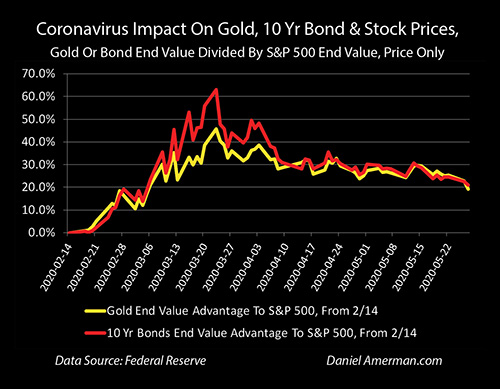It is also why, as explored in my most recent analysis (link here), long bonds were strongly outperforming gold when the stock market was bottoming out in March, because so many more institutional investors were crowding in and trying to get a piece of the profits that the Fed creates when it fights crisis via interest rates and bond purchases.

### Crossing The Zero Percent Barrier

What we went through above are the bedrock mathematical foundations of investment pricing and financial analysis. The future value equation, and its inverse, the present value equation, underlie most of finance and investments, and (in theory), variations of those equations drive not only bond prices but everything from stock prices to real estate prices to retirement financial planning to how pensions are funded to mortgage amortizations and to how insurance premiums are calculated.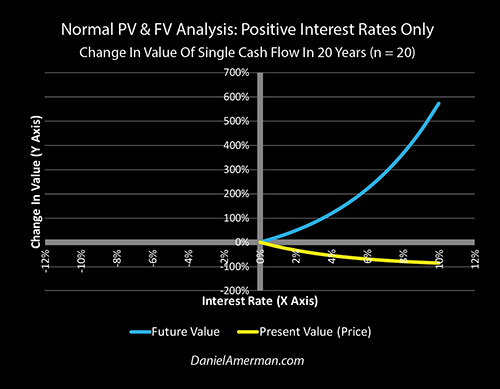When we look them in terms of XY coordinates, then we get what is plotted out in the somewhat unusual graph above, which shows changes in future values and changes in present values for a cash flow 20 years out, with increasingly positive interest rates. The higher the interest rate, the greater not only the interest but the compounding of interest over 20 years, and the blue line of changes in future value curves upwards (aka positive convexity).

The higher the interest rate, the less the investor will pay for the future cash flow today, and the greater the discount required, as shown with the yellow line of changes in present value.

Now, there is nothing unusual about plotting these relationships on a graph, but what would have been deeply weird, at least until relatively recently, is to have that big blank area on the left, allowing for increasingly negative interest rates. (The presentation is also a little unusual in that it shows changes in future value and changes in present value, instead of just future value and present value, so we get the intersection of the lines and the division of the quadrants at the (0,0) origin point for reasons that should become clear below.)

Nobody did this for a very long time because negative interest rates are profoundly unnatural, indeed one could call them a perversion of the normal financial order. No rational investors in a free market without central banking interventions would even dream of voluntarily buying investments with negative interest rates, so there was no need to have graphs that crossed the 0% line in the middle and continued into the bizarre territory of increasingly negative interest rates.

However, as it turns out the perversion of the natural financial order is the current order of the day, and we have the Bank of Japan, the European Central Bank and now the Bank of England all effectively an using an unnatural process of monetary creation to buy securities at prices higher than any rational investor would pay (absent such interventions), and are thereby creating negative interest rates through the process explained in more detail in Chapter 14 (link here).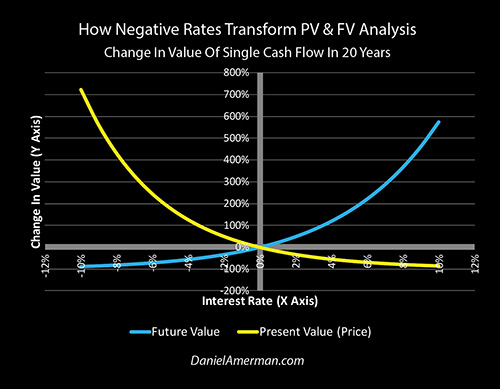When we complete the left side of the graph for the negative interest rates that have been forced on much of the world, then from a mathematical perspective we get the fairly clean and simple graph above, of two smoothly changing lines, each continuous, with an intersection at the origin point of 0% interest rates and 0% changes in value. There is nothing special or particularly interesting about it for a mathematician, it is pretty vanilla stuff.

However, from a finance and investments perspective, we get a complete inversion of the natural order that is so deeply weird and bizarre that it can be hard to wrap your mind around it.

We also get a double anomaly that the United States hasn't seen before, but which would create extraordinary profits in a very short time period. Indeed, what the lines show us is:

1) for a given change in rates, how to create more wealth than would be created by the magic of compound interest over 20 years; and

2) how to do so instantly, up front, instead of having to wait 20 years for the interest payments to compound.

To get to the secret of those unprecedented and potentially explosive profits, we just have to do a little simple math, which can again be done on your phone or calculator (and this is also why I went through the examples and equations to get to this crucial point).

### Two Lines, Four Quadrants, Explosive ProfitsTo understand the double anomaly which can create more money in a fraction of the time, I've labeled the four quadrants in the graph above, and have also put in the numbers for four symmetrical points, so we can see 4 concrete examples of what is happening and why. (This use of -6% versus +6% develops the relationships with a clear and symmetrical example, that can then be applied to less negative rates, such as -1%.)

1) The upper right quadrant is the normality of how wealth grows over time with interest and the compounding of interest at steadily higher rates, it is future value with positive rates.

2) The lower right quadrant is the normality of how the price paid for an investment decreases with steadily higher positive rates, it is present value with positive rates.

3) The lower left quadrant is the deep abnormality of how wealth steadily decreases over time when invested at negative interest rates, it is future value with negative rates.

4) The upper left quadrant is the still deeper abnormality of how the price of an investment shoots upwards as rates become steadily more negative, it is present value with negative rates.

Point A is the future value of an investment with 6% interest rates for 20 years - and the gain is 221%. However, it does take 20 years to earn that full 221% gain.

Note that future values with positive rates are an unbounded exponential series, where wealth can grow exponentially without limits, given high enough investment rates, and/or the passage of enough time and the accompanying growth in compound interest.

(The numbers are ((1+i)^n), (1+.06)^20) = 321%, 321% future value - 100% starting value = 221% in future interest earnings, or change in value.)

Point B is the price today of a cash flow 20 years out, with a 6% yield. To get that yield, an investor would need a 69% discount, meaning that they would be willing to pay 31 cents on the dollar. That is -69% change in value, relative to the 0% discount involved in buying a cash flow 20 years out with a 0% yield - i.e., paying full price.

(The numbers are 1/((1+i)^n), 1/((1+.06)^30)), 1 / 3.21 = 31%, 100% - 31% = a minus 69% change in value.)

Restated, while how prices are determined can mystify many average investors - it's just a ratio. We already did the real work of running the exponential mathematics to determine that \$1 would be become \$3.21 in the future value equation. Once we have those two numbers, then determining price is just simple arithmetic. Dividing \$1 by \$3.21 means that if we pay 31 cents on the dollar, for a dollar that will be received in 20 years, we will have locked in a 6% yield for the 20 years. So, that's the price we pay, 31 cents on the dollar or a 69 cent discount, and we get the 6% yield.

Once that's understood - then many things about how the Fed has changed stock, bond and real estate prices can be understood, just by looking at the right side of the XY graph. The lower that the Fed forces interest rates - then the less "compound interest" or future value that is created, and the less future wealth there is for average savers or for investors following many traditional long term investment strategies. However, the less the future compound interest, then the lower the number that we divide by in order to get price today.

So (all else being equal), the lower that the Fed pushes interest rates in order to contain crises such as the pandemic economic shutdowns or the crisis of 2008, then once economic stability is restored, the higher the prices a rational investor will pay today for not just bonds, but also stocks and real estate (which is a key part of the book). The closer we get to zero percent, then the greater the crippling of future compound interest, and therefore the greater the magnitude of the price increases today relative to historical norms.

Note also that when we take the inverse of the unbounded exponential series, that of future value, we get a bounded series, so long as we have positive interest rates. We would never pay more than 100 cents on the dollar. We never reach zero either, the discount never reaches -100%, though the higher the rate and/or the longer the term, the closer we get to zero.

Point C is the value of our investment 20 years in the future, when we buy it at a negative yield of 6%. To see how that works, let's go back to our equation.

To get future value for a positive rate, we simply add the rate to 1, compound for the number of years, and multiply times our starting investment. To get future value for a negative rate - we simply add a negative rate to 1, compound for the number of years, and multiply times our starting investment. So instead of raising 100% plus 6% to the 20th power, we raise start with 100%, subtract 6%, and raise the 94% that remains to the 20th power. Every time we multiply a number that is less than one times itself, the smaller the number we get, and if do that 20 times with 94%, we end up with 29 cents on the dollar, meaning our change in value is minus 71%, as shown at Point C.

(The numbers are ((1 + i) ^ n), (1 + -.06) ^ 20) = (1 - .06) ^ 20) = (.94 ^ 20) = 29%, 29% future value - 100% starting value = -71% change in value.)

Restated, this isn't difficult, once we take that huge first step of going to bizzaro-land - as the Bank of Japan and European Central Bank already have - and look at central banks using both monetary creation and their banking authority to force negative interest rates on a financial system.

How do we get a negative 6% annual yield for one year? Simple, we pay \$100 for the privilege of getting \$94 paid back to us one year from today. That locks in the \$6 loss on our initial \$100 investment, which is negative 6%.

To get a negative loss of 6% per year for two years, we would multiply 94% times 94%. So, we pay \$100 today for what would be \$94 a year from now, but then we multiply times 94% again to get our 6% loss for the second year, and the actual number is \$88.36.

Do that 20 times in a row, and we invest \$100 today, for the privilege of receiving \$29 in the future. That locks in an annual loss of exactly 6% per year for the next 20 years. When we take inflation into account, then the real value of that \$29 is likely to be much lower, and our real annual losses in inflation-adjusted terms are going to be worse than -6%.

(This is also a good place to note that these are simplified numbers. Actual bond prices will be based on Bond Equivalent Yields, meaning semiannual compounding instead of the annual compounding used herein, and we also have to look at things such as coupons and settlement dates. This analysis is intended to conceptually present the relationships, without getting bogged down in the details.)

Note that when we cross the 0% rate barrier and go to negative rates, then as can be seen in the graph repeated above, the blue line of our unbounded exponential series becomes a bounded series. When we multiply a positive number that is less than one by itself, such as 1 - .06 = .94, it can never reach 1, but it can never go below zero either.

So, the blue future value line in the lower left quadrant has transformed to look a lot like the yellow present value line in the lower right quadrant, but it really isn't. First, it is slightly more negative, which becomes important with negative rate prices. More importantly, they are in different times. The entire blue line of future values exists 20 years in the future, the yellow line of present values is the prices (and price changes) that exist today. So it would take 20 miserable years of losing 6% per year to knock us down to 29%, and to have lost the full 71% of the starting value (and that is before the further losses associated with inflation).

Point D is the strangest and least understood point on the graph - and also possibly the most profitable.

Returning to our present value equation, we once again subtract 6% instead of adding 6%, if we have a negative 6% yield instead of a positive 6% yield. So once again, we are raising 94% to the 20th power as we did with future value, but remember - present value is the inverse of future value. So we divide 1 by (.94 ^ 20), and we get 345%. That means that we have a 245% gain, as shown with Point D.

(The numbers are 1 / ((1 + i) ^ n), 1 / ((1 + -.06) ^ 20)), 1 / (.94 ^ 20), 1 / .29 = 345%, 345% - 100% = a positive 245% change in value.)

Restated, this is again simpler than it looks, once the mind-blowing perversion of the natural financial order that is negative interest rates is accepted. As with positive rates, we know the ratio of what we have to invest up front in order to get a given amount of cash in the future. With the future value equation we established that we have to invest \$100 to get \$29 in order to earn a -6% annual yield for 20 consecutive years. Because 100/29 = 3.45, we have to invest \$3.45 for every \$1 we get back if we are to lock in a 6% loss each year for 20 years.

This means that the dollar price for a \$100 bond is therefore \$345, or 345%, of which \$100 is the par value of the bond that we will be paid in 20 years, and \$245 is the premium that we pay today (or get paid by a buyer today) for the privilege of locking in those losses at that rate. It is the planned loss of the entirety of that \$245 premium for an investment held to maturity that creates the negative rates. It is also the payment of that huge premium to a seller, who bought at positive rates and sells at negative rates, that creates the extraordinary profits.

It is only when we complete our fourth sample data point, and walk through the bizarre mathematics of the fourth quadrant, that we can discover the unnatural but extraordinary extent of the profits that can created by the artificial and unnatural financial state of negative interest rates. Other central banks have already been forcing those rates onto their financial systems and they may yet be coming to the United States, as the Federal Reserve tries to find ways to pull the U.S. economy out of the pandemic shutdowns recession.

We can see another seeming mirror relationship when the 0% barrier is crossed, with the yellow negative rate present value line looking a lot like a mirror of the blue positive rate future value line. However, it really isn't the same at all, and the differences are critical.

1. Compound interest has been called the Eighth Wonder Of World, and has been described as one of the most powerful forces in the universe. The exponential mathematics driving compound interest have made it the single most reliable long term creator of wealth in financial history, whether we are talking about bonds and reinvested interest payments, or stocks and reinvested dividend payments.

However, when we take the inverse of the exponential destruction of wealth, which is the inverse of not gaining 6% per year, but of losing 6% per year - then we get something more powerful than compound interest. Compare the symmetrical numbers. With Point A, and investing with a 6% increase in rates (6% instead of 0%), there is a 221% gain. With Point D and pricing in the effects of a 6% decrease in rates (-6% instead of 0%), there is a 245% gain.

The present value price gains from further lowering negative interest rates and accelerating the destruction of wealth, exceed the future value compound interest gains from increasing positive rates and accelerating the creation of wealth, all else being equal.

2. The blue line of future value is 20 years in the future in the above graph. It takes 20 long years to gain 221% via the power of compound interest with a positive 6% rate, and it take 20 long years to lose 71% with a 6% negative rate.

The yellow line of present value is right now, today, there is no delay. So, and this is a simplified example - but the math works - if rates were to move from 0% to negative 6% in a single day, then the entire 245% gain in price would also occur in the same single day, with no need to wait a year, let alone 20 years.

So yes, more profits than compound interest, and delivered up front instead of having to wait 20 years.

3. When we go to the left with the yellow line of present value, the rate at which we earn price profits tilts ever more sharply upwards (aka positive convexity). Indeed, this remains continuously true as we travel along the yellow present value line from the lower right to the upper left, going from high positive interest rates to low positive interest rates to zero percent interest rates to low negative interest rates to deeply negative interest rates.

The move from 0% interest rates to negative 1% interest rates will produce greater price profits than was ever seen with any 1% changes in yield with positive interest rates. And if rates were to move from negative 1% to negative 2%, that would produce greater price profits than the move from 0% to negative 1%. Every further move deeper into negative territory creates a new record price gain, that is that much greater than any of those ever experienced with positive interest rates.

Again, we can see a rough mirror relationship with the blue line of compound interest and future value, but not quite. The yellow line of price changes produces higher gains, those gains increase faster the farther away we get from 0%, and - they are all realized up front.

### Part Two

This is the end of Part One of this analysis.  In Part Two, we will:

Examine the profits associated with a move to negative 1% yields.

Look at the impact of time, and how prices change with 10 year and 30 year bonds, instead of 20 year bonds.

Identify where the money comes from to buy bonds at such unnatural prices.

Look at where the profits are likely to go, and how a move to negative rates creates an unfair redistribution of wealth, where most of the profits go to Wall Street and major institutional investors, while smaller investors suffer.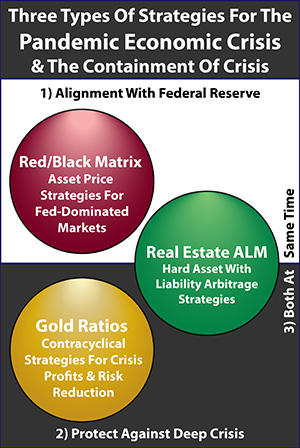Discuss the positioning of buying bonds in anticipation of negative rates among the three basic categories of investment strategies for the pandemic economic crisis and containment of crisis.

Daniel R. Amerman, CFA

Website: http://danielamerman.com/

Daniel R. Amerman, Chartered Financial Analyst with MBA and BSBA degrees in finance, is a former investment banker who developed sophisticated new financial products for institutional investors (in the 1980s), and was the author of McGraw-Hill's lead reference book on mortgage derivatives in the mid-1990s. An outspoken critic of the conventional wisdom about long-term investing and retirement planning, Mr. Amerman has spent more than a decade creating a radically different set of individual investor solutions designed to prosper in an environment of economic turmoil, broken government promises, repressive government taxation and collapsing conventional retirement portfolios